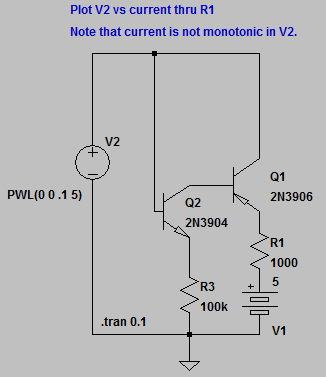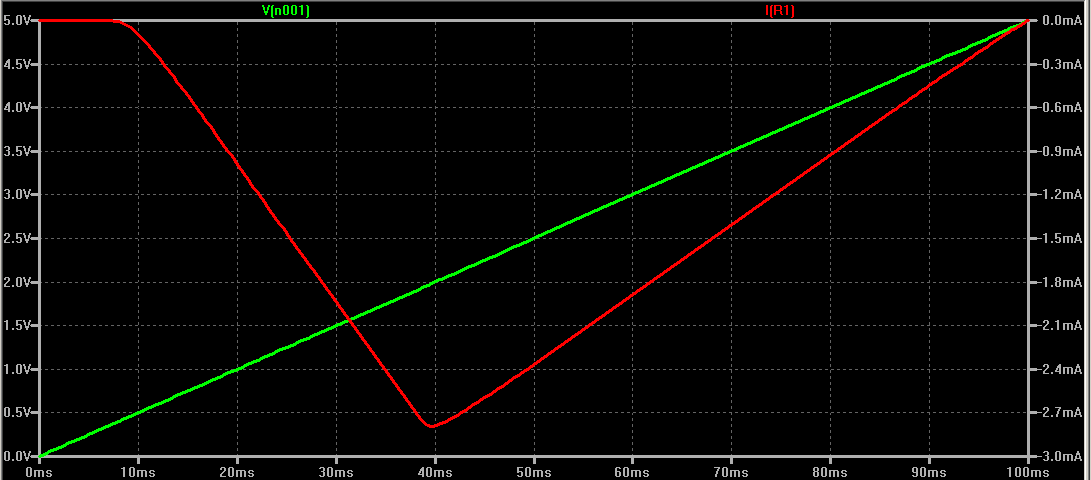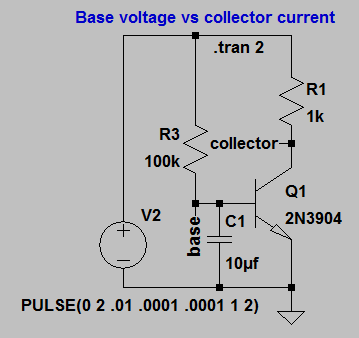## ECE 1810 (ENGRI 1810) Homework 3

1. Download the LTspice circuit transistor model of Na channel and run the simulation. Look at the waveforms for V2 and the current through the collector of the 2N3906. Compare to the IV curve for a real sodium channel. Notice that for both, as voltage increases there is a dip in current(corresonding to a negative resistance) followed by an increase. A negative resistance always requires external energy to force charges to go the wrong way. In the case of the souium channel, the enery comes from a large sodium concentraion gradient pushing ions against the electical gradient. For this circuit the energy comes from the 5 volt battery.
1. What happens to the shape and position of the current curve as you change R1?
2. Use LTspice to compute `V(n001)/I(R1),` (which is the effective resistance of the circuit from V2 to ground) by making sure the waveform window is active, then using the menu item PlotSettings>Add Trace and fill in the dialog box. Is the effective resistance approximately the same as R1 for some values of R1? Try at least 200 ohms, 1000 ohms, and 5000 ohms. Is the effective resistance constant over the voltage range? You will need to limit the voltage range so that the current never goes to zero, because dividing by zero overflows the computation.2. Download the LTspice circuit transistor model of an NPN transistor circuit used to model the potassium channel. Run the simulaton and plot the pulse input, the base voltage and the collector current. Notice the RC time delay at the beginning of the pulse before current starts flowing.
1. Explain the time delay quantitatively by computing the time it takes the function `Vbe=V2*e(-t/R3C1)` to reach the turn on voltage (about 0.6 volts) of the transistor.
2. Doubling V2 should therefore cut the time in half. Does it? Doubling C1 or R3 should double the time. Try it.
3. If the transistor is completely on, then the current should be approximately V2/R1. How close is the current to V2/R1 for V2=2 and V2=4 volts?September 19, 2011 Copyright Cornell University